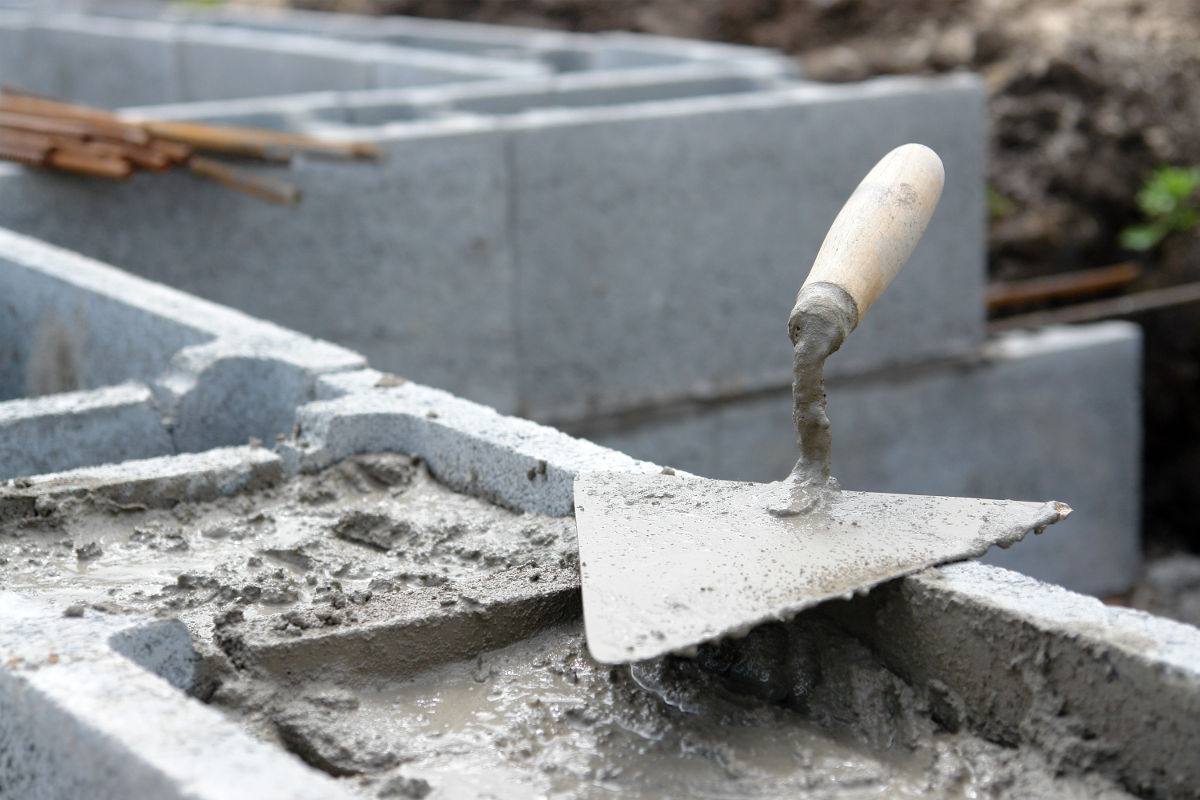# Concrete Block Fill Calculator – Find CMU Fill Volume

Find the core fill volume for a concrete block wall and estimate how much grout is required to fill the block using the width and height of a wall or given a number of CMUs to fill.

## Estimate Fill Using Wall Dimensions

Optionally enter the price per cubic yard
\$

## Estimate Fill Using Number of 16" x 8" Blocks

Optionally enter the price per cubic yard
\$

## Material Estimate:

Grout Needed

Cubic yards

80lb Bags
Material Cost

Estimated cost
Learn how we calculated this below

## How to Find Concrete Block Core Fill Volume

Concrete block, also known as a concrete masonry unit and sometimes called a cinder block, typically has one or two holes or voids to reduce the weight of the block. To estimate how much grout is needed to fill those voids you need to find the volume of each void and multiply that by the number of voids in the wall.### Step 1: Find the Volume of Each Block Void

Start by measuring the length, width, and height of the holes in the block in inches. Then multiply those dimensions together to find the volume in cubic inches. Use our volume calculator to simplify this process.

### Step 2: Find the Number of Voids in the Wall

The next step is to find the number of voids in the wall. This can be done by counting the number of blocks in the wall. Use our concrete block calculator to find how many blocks are in your wall. If each block has two voids then multiply the number of blocks by 2 to get the number of voids in the wall.

### Step 3: Find the Total Concrete Block Fill Volume

Now, multiply the number of voids by the volume of the void in cubic inches to find the total grout fill volume in cubic inches. Divide the final number by 46,656 to find the volume in cubic yards. A conversion calculator can make this easy.

## Fill Volume for Various Block Wall Thicknesses

Approximate grout fill volume and coverage for a concrete block wall
Block Wall Thickness Blocks Filled per Cubic Yard Concrete/Grout per 100 Block Concrete/Grout per 100 Square Feet Wall Area
6″ 120 .83 yd3 .93 yd3
8″ 100 1.0 yd3 1.12 yd3
10″ 80 1.23 yd3 1.3 yd3
12″ 65 1.54 yd3 1.73 yd3